This page discusses some of the issues that come up in numerical circuit simulation for the Virtual Crossover.

I wanted to be able to handle fairly general circuit configurations, including for example magnetic coupling in inductors. Since I have had some experience in circuit simulation, I decided to write my own program, rather than trying to use an existing package such as SPICE. This was partially motivated by the fact that I needed to do some of the analyses in the frequency domain, as I will explain elsewhere, and it wasn't clear to me how to do this with SPICE, although it may be possible.

Even after deciding to do a general numerical analysis, there are still some issues that remain. As an example, consider the following high-pass filter circuit: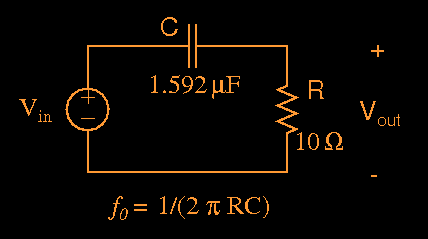Figure 1: High-pass filter example with f0=10kHz.

This is not meant to be a realistic example, but it does illustrate some of the problems involved in modeling circuits. In Fig. 1 you can think of the capacitor as the crossover circuit and the terminating resistor as the driver; the input Vin would be the audio signal coming from the amplifier, and Vout would be the signal at the driver terminals. The corner frequency for this circuit is 10 kHz.

The method I use to characterize crossover circuits is to calculate their impulse response. Even though the circuit of Fig. 1 is quite simple, it does present a challenge since it is not band limited, i.e. all frequencies above f0 are passed to the output. Most of the techniques used in digital signal processing require that waveforms are band-limited, otherwise aliasing will occur and the results will not be accurate.

First, consider the ideal impulse response of this high-pass filter. To obtain the impulse response, a Dirac delta function, denoted by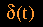is impressed at the Vin terminals. The functionis of infinite magnitude and vanishingly small duration, but such that its time integral is unity. In other words,can be thought of as having magnitude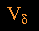lasting for a time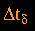, such that, and where the limit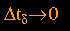is taken. Formally, the impulse response of this high-pass filter at the Vout terminals is given by: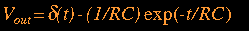(1)

We can almost guess the result in Eq.(1) by considering what happens when an impulse is applied. Since the impulseis infinite and since the voltage on a capacitor can't change instantaneously, the impulse is passed through to the resistor nearly unchanged; this accounts for the first term in Eq.(1). Also during the time the impulse is applied, current of an amount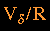Amps flows into the capacitor, for a time. Therefore at the end of the impulse, the total charge on the capacitor will be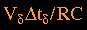, or. The polarity of this capacitor voltage will be such that when the impulse is removed, it will produce a negative voltage at the output terminals of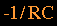Volts. Thereafter, the voltage at the output will decay exponentially.

The thing that causes the problem is basically thefunction in Eq.(1). Obviously in any real numerical calculation we will not have a function that is infinitely large and exists for an infinitely short time. So the question is, how can we come up with a good approximation for the impulse response of Eq.(1)? And it has to be done in a general way, we can't cheat and use the fact that we already know the formal impulse response for this circuit.

One method that seems quite plausible is to use the discrete time impulse function,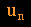, as the excitation at the Vin terminals. If we are doing a calculation at discrete points separated by time step, the functionis equal to 1 during the interval ofseconds denoted by index 0, and it is equal to 0 otherwise. In fact, the functionis often described as the discrete-time equivalent of the impulse functionfor continuous-time systems.

I have written a program called IMPRSP.C, and one of its options is to apply a value of 1 at the circuit input for one time step, and then 0 for the remaining simulation time. Fig.(2) shows a portion of the resulting simulation for the circuit of Fig.(1):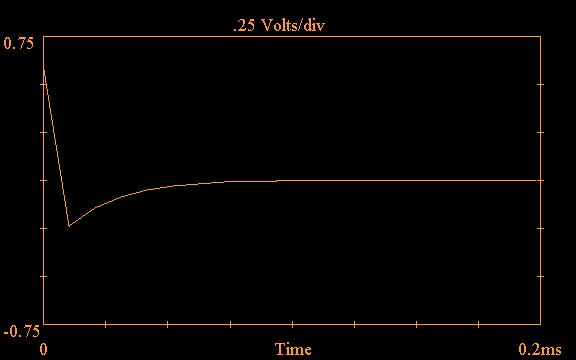Figure 2: Impulse response for the circuit of Fig.(1) using the discrete-time impulse function.

In this simulation, the sampling rate is 96kHz, however the IMPRSP.C program simulates the circuit at a much smaller time step in order to produce more accurate results; output values are only saved at the 96kHz sampling rate. Also, note that values in Fig.(2) are only shown at discrete points, i.e. I don't show values as constant within the duration of the 96kHz time step.

One thing to notice in Fig.(2) is that the first point where the impulse is applied is wrong; we expect it to be 1, but it is smaller than 1, specifically it comes out to be about 0.604. This is because values in Fig.(2) are stored at the end of each time step, and during the time that the impulse function is applied the capacitor is charging up, so that the output voltage across the resistor is reduced from 1. You might think that we could fix this by saving the value at the beginning of the time step instead, however it turns out that if we were simulating a low-pass filter instead, the more accurate thing would be to store the value at the end of the time step, just as we have done in this case. So we can't use that as a general fix. Basically, this is an illustration of the kinds of ambiguities that arise when we try to model a system that is not inherently band-limited using digital sampling techniques.

The abrupt jump to the second waveform point in Fig.(2) occurs when the discrete impulse function is removed. It turns out that this value is in error also, partially because the initial value at the beginning of the time step is not right as discussed above, and also because it takes some time to simulate the second point (the sampling time), after which the output voltage has changed. Nevertheless, the general shape of the waveform in Fig.(2) is correct, and it displays the general properties of the true impulse response; also, it doesn't display any ringing in the time domain that is often observed in digital signal processing results. So the question arises, can we use this method?

One way to answer this question is to take the FFT (Fast Fourier Transform) of the waveform in Fig.(2) and compare with the expected high-pass frequency characteristics; after all, we know the exact solution for the circuit in Fig.(1). The exact solution for the output voltage magnitude as a function of frequency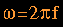is: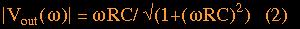Fig.(3) shows the magnitude of the frequency response out to 20kHz calculated from the FFT of the time domain waveform in Fig.(2):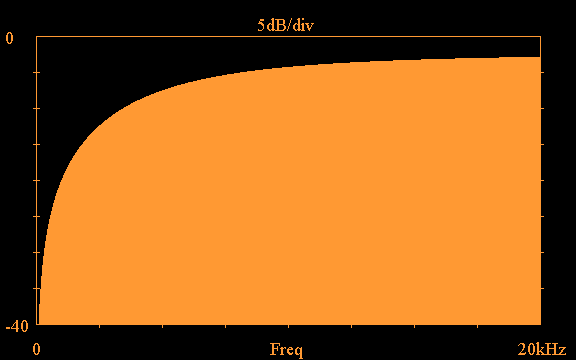Figure 3: Magnitude of the frequency response of Fig.(2) using the discrete-time impulse function.

The frequency response certainly looks good and shows a high-pass characteristic; it is only through careful inspection of the numerical results that problems appear. For example, from Eq.(2), the frequency response at the corner frequency of 10kHz should be down by 3.01dB; however examining the data corresponding to Fig.(3), one finds that the calculated result at 10kHz is down by 4.3dB. At 20kHz, Eq.(2) says that the response should be down by 0.969dB, however the calculated result is down by 2.89dB at 20kHz. Obviously this method cannot be used if we want to accurately predict the response of crossover circuits.

The method that I have described thus far is basically what is known as the "impulse invariance" technique of digital filter design . This method starts with the time-domain impulse response of an analog filter and attemps to design a digital filter with the same time domain response at its discrete sample points. Oppenheim and Schafer  come to the following conclusion regarding this method: "...the impulse invariance technique is obviously only appropriate for essentially bandlimited filters ... highpass or bandstop filters would require additional bandlimiting to avoid severe aliasing distortion."

One way around this problem, which is indicated in the above quote, is to apply a band-limited signal at the Vin terminals, instead of a non-band-limited impulse function. That way, even if the circuit itself is not band-limited, the calculated response will be. I outlined this technique in a previous article , where I used an equal-ripple lowpass filter as the band-limited input. I have written a program called ERLPF.C that can generate such a waveform. For example, Fig.(4) shows the time-domain impulse response of an equal-ripple lowpass filter with passband edge at 30kHz and stopband edge at 42.64kHz: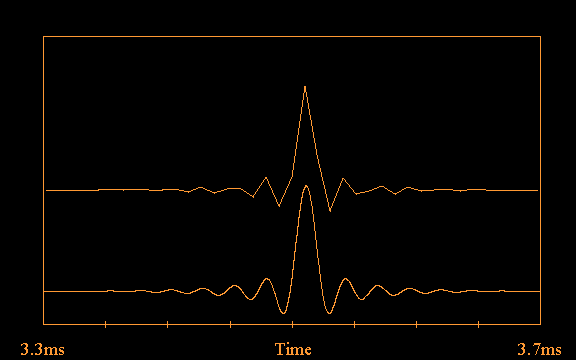Figure 4: Impulse response of 30kHz equal-ripple lowpass filter sampled at 96kHz (top trace) and 4800kHz (bottom trace).

For this particular filter, the stop-band ripple is down by 120dB, so it is very effective at band-limiting. Note that the time-domain response has been shifted about 3.5ms in time; as originally calculated it is symmetric about t=0, but to use it in a simulation it must be shifted up in time until the ringing in the waveform is sufficiently small at t=0. The top trace of Fig.(4) shows the filter response sampled at 96kHz; this may seem to be a crude representation of the waveform, but according to sampling theory we only need to sample at a rate greater than twice the maximum frequency content of the signal. Since the waveform in Fig.(4) is 120dB down at 48kHz, a sampling rate of 96kHz is sufficient. We can recover the details of the waveform using sin(x)/x interpolation ; the lower trace in Fig.(4) shows the result of re-sampling the upper trace at 50 times the sampling rate, using sin(x)/x interpolation to fill in the intermediate points.

Figure 5 shows the magnitude of the filter response in the frequency domain: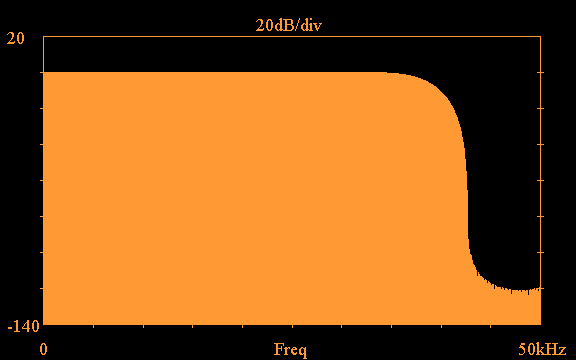Figure 5: Frequency response magnitude for the equal-ripple lowpass filter of Fig.(4).

We can use the IMPRSP.C program to simulate the circuit using the lowpass filter of Fig.(4), since one of the program's options is to accept an arbitrary input waveform to the circuit. In order to carry out an accurate simulation, IMPRSP.C can resample the input waveform using sin(x)/x interpolation, as was done in the lower trace of Fig.(4); that way it can calculate the simulation at a much shorter time step than the final sampling rate at which results are stored.

Figure 6 shows the result of carrying out the IMPRSP.C calculation for the high-pass circuit of Fig.(1) when the equal-ripple lowpass filter of Fig.(4) is used as the input to the circuit:Figure 6: Impulse response of the high-pass circuit of Fig.(1) calculated using the program IMPRSP.C with input waveform the lowpass filter of Fig.(4).

This waveform certainly doesn't look much like what we would expect based on the exact solution given by Eq.(1); however, the basic features of the solution can still be recognized. The localized delta functionis replaced by the band-limited impulse response of Fig.(4). And you can see that to the right of the waveform peak, it shifts downward and gradually recovers toward zero, as does the ideal solution. But the real test of Fig.(6) is how closely its frequency response characteristics approach the correct values. Figure 7 shows the results of taking the FFT of the waveform of Fig.(6):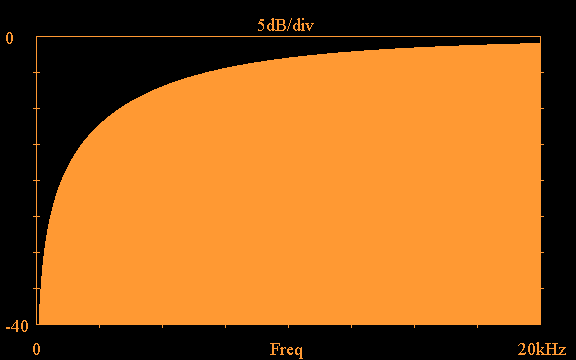Figure 7: Frequency response magnitude from FFT of the waveform in Fig.(6).

Comparison of the calculated data at 10kHz and 20kHz shows that this method yields the correct frequency response magnitude to within 3 decimal places. So it appears that time domain simulation with band-limited input can be used to accurately characterize the behavior of crossover circuits.

Even though excellent frequency response agreement is obtained with this method, I am bothered by the introduction of excessive ringing in the time domain to the waveforms. This ringing is essentially at the cutoff frequency of the lowpass filter that is used, in this case in the 30-40kHz range, so it is not within the audio band and may not be important. But an important criterion for me has always been achieving good time domain response in speaker systems, so introducing so much ringing goes against my grain. Unfortunately, at this point I don't see a way around it - we need to band-limit the signal to use digital signal processing techniques, and if we are to use a practical sampling rate such as 96kHz, the signal needs to be band-limited somewhere between 20kHz and 48kHz, which calls for a filter with a sharp cutoff, and which necessarily exhibits ringing in the time domain. Oversampling at a much higher rate and using a gentler filter would certainly help, but if we want to eventually get back down to 96kHz, we still need to be band-limited at 48kHz. If anyone knows of a solution that would eliminate this ringing, I would be very interested to learn about it.

Before leaving this topic I want to mention one additional simulation technique that can be used, which yields essentially identical results to the time-domain simulation approach; you can also carry out the simulation in the frequency domain. Imagine decomposing Vin into its frequency components, so that it becomes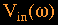; then the circuit can be solved for each frequency point, yielding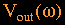, which can finally be transformed back to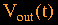. I wrote another program, IMPRSPZ.C, which does such a frequency-domain calculation; this program assumes that the circuit is terminated with a known impedance as a function of frequency,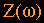. I will discuss this method further when coupling the circuit and driver is discussed. The frequency-domain method is actually much faster than the time-domain simulation with IMPRSP.C, because there is no need to over-sample in order to obtain an accurate solution.

References

 Oppenheim, Alan V., and Schafer, Ronald W., Digital Signal Processing, Prentice-Hall, Inc., 1975.

 Mains, Richard K., "The Virtual Crossover, Part 1", AudioXPress, May 2001.

Home